# Binary Division & Multiplication: Rules & Examples

An error occurred trying to load this video.

Try refreshing the page, or contact customer support.

Coming up next: Four Basic Arithmetic Operations for Floating Point Numbers

### You're on a roll. Keep up the good work!

Replay
Your next lesson will play in 10 seconds
• 0:03 Binary Multiplication
• 2:09 Binary Division
• 4:19 Lesson Summary

Want to watch this again later?

Timeline
Autoplay
Autoplay
Speed

#### Recommended Lessons for You

Lesson Transcript
Instructor: Sebastian Garces

Sebastian has taught programming and computational thinking for University students and just got accepted at PURDUE for Master's degree

Binary numbers are the basis for all that is happening inside computers or electronic devices. In this lesson, learn how to multiply and divide binary numbers.

## Binary Multiplication

Binary division and multiplication are both pretty easy operations. Instead of dealing with a lot of numbers, you just need to make sure to set the 1 or 0 in the right place. For this reason, you need to make sure you are also familiar with binary addition and subtraction.

To perform a binary multiplication problem, we need to understand how addition works with binary numbers and follow the same process of multiplication and addition we would use with decimal numbers.

Let's say we want to multiply 4 by 3, which in binary will be 100 by 011 (or 11, it's the same):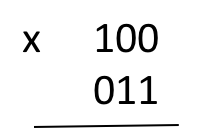We start with the first digit, in this case 1, and multiply 100 by 1, which gives the exact same number.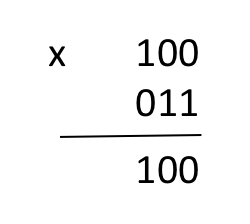Then we move to the second digit and proceed to do the same. Since it's also 1, the number will remain intact, we just need to shift the number one digit to the left: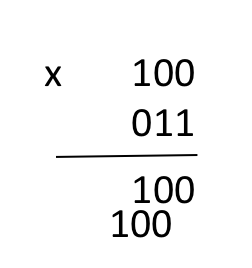Later, since the last digit is a 0, we can skip it and start with the addition: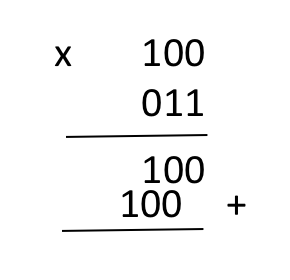0 added to 0 is 0. And, 1 added to 0 is 1: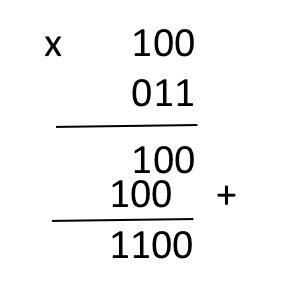We can verify that the result is 12 by converting the binary product to decimal.

Now, let's try with a harder example. Let's multiply 12 by 15, which in binary will be 1100 by 1111.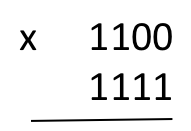First, you take the first digit from 1111 and multiply it by 1100, which gives the same number: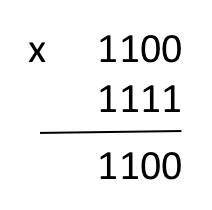Now we're going to repeat the same process with each digit, shifting to the left with each of them, getting this descending series of 1100s: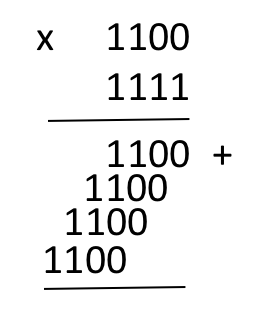Finally, we just need to add the numbers. This can be a little tricky when you add several 1's in a row.

Once we reach the column with the two consecutive 1's, remember that the result is 0 and we carry a 1 to the next column.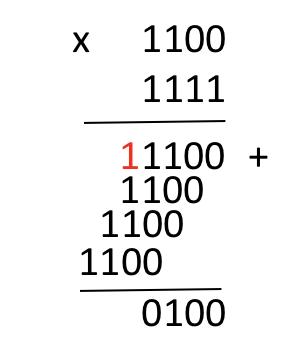In the next column, we need to add the numbers by pairs. The first two 1's will add up to 0, carry a 1, then, the result 0 plus the 1 will add up to 1.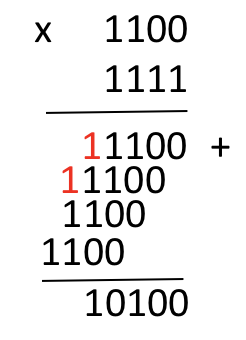We keep repeating the same process until we finish all the addition: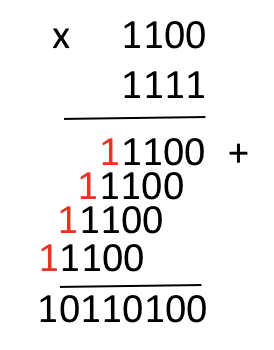We can check that 12 multiplied by 15 is 180, which in binary is 10110100.

## Binary Division

To perform a binary division, we need to follow the same process as we do for dividing regular numbers but, in this case, we only need to decide if it's going to be a 1 or a 0.

To divide two numbers, which result is an exact division, we basically need to follow four steps: division, multiplication, subtraction, and next digit.

Let's say that we want to divide 18 by 3, which in binary will be 10010 divided by 00011 (or 11, it's the same).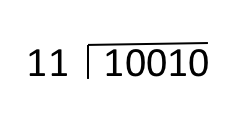First, we need to identify digit by digit. Is 11 less or equal than 1? No, so we get another digit. Is 11 less or equal than 10? No, so we repeat and get another digit. Is 11 less or equal than 100? Yes, so in this case we don't need to think how many times can 100 be divided by 11, instead, we just add a 1 to the product.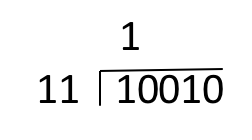To unlock this lesson you must be a Study.com Member.

### Register to view this lesson

Are you a student or a teacher?

### Unlock Your Education

#### See for yourself why 30 million people use Study.com

##### Become a Study.com member and start learning now.
Back
What teachers are saying about Study.com

### Earning College Credit

Did you know… We have over 160 college courses that prepare you to earn credit by exam that is accepted by over 1,500 colleges and universities. You can test out of the first two years of college and save thousands off your degree. Anyone can earn credit-by-exam regardless of age or education level.